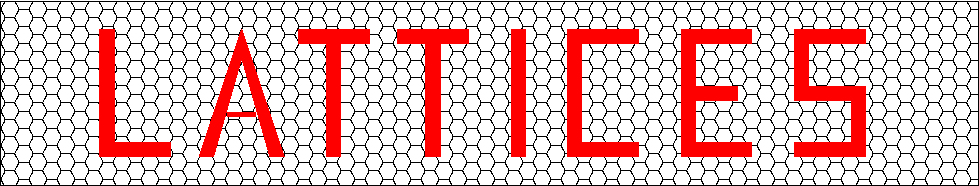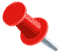## Table of Densest Packings Presently KnownKeywords: tables, density, center density, lattices, quadratic forms, packingsPart of the Catalogue of Lattices which is a joint project of Gabriele Nebe, RWTH Aachen university (nebe@math.rwth-aachen.de) and Neil J. A. Sloane, (njasloane@gmail.com).Densest packings presently known in dimensions up to 128.
The entry gives the densest lattice known
(and if a denser nonlattice is known this appears in parentheses)
For further details about any of the packings mentioned that don't have links, see SPLAG 3rd edition

DimCenter density for lattice (nonlattice)Lattice (resp. nonlattice)
11/2 = 0.50000LAMBDA1 = A1 = Z
21/2 sqrt 3 = 0.28868LAMBDA2 = A2
31/4 sqrt 2 = 0.17678LAMBDA3 = A3 = D3
41/8 = 0.12500LAMBDA4 = D4
51/8 sqrt 2 = 0.08839LAMBDA5 = D5
61/8 sqrt 3 = 0.07217LAMBDA6 = E6
71/16 = 0.06250LAMBDA7 = E7
81/16 = 0.06250LAMBDA8 = E8
91/16 sqrt 2 = 0.04419LAMBDA9
101/16 sqrt 3 = 0.03608 (5/128 = 0.03906)*LAMBDA10 ( P10c )*
111/18 sqrt 3 = 0.03208 (9/256 = 0.03516)*KAPPA11 ( P11a )*
121/27 = 0.03704KAPPA12 = K12 (the Coxeter-Todd lattice)
131/18 sqrt 3 = 0.03208 (9/256 = 0.03516)*KAPPA13 ( P13a )*
141/16 sqrt 3 = 0.03608LAMBDA14
151/16 sqrt 2 = 0.04419LAMBDA15
161/16 = 0.06250LAMBDA16
171/16 = 0.06250LAMBDA17
181/8 sqrt 3 = 0.07217 (39 / 49 = 0.07508)*LAMBDA18 (B18 [BierEdel96])*
191/8 sqrt 2 = 0.08839LAMBDA19
201/8 = 0.12500 (710 / 231 = 0.13154)*LAMBDA20 (B20 [Vard96])*
211/4 sqrt 2 = 0.17678LAMBDA21
221/2 sqrt 3 = 0.28868 (0.33254)*LAMBDA22 (R22 [CoSl96])*
231/2 = 0.50000LAMBDA23
241Leech lattice LAMBDA24
251/ sqrt 2 = 0.70711LAMBDA25
261/ sqrt 3 = 0.57735LAMBDA26, T26
271/ sqrt 3 = 0.57735 (1/ sqrt 2 = 0.70711)*B27 [Bace96] (B*27 [Vard96a])*
282/3 = 0.66667 (1)*B28 [Bace96] (B*28 [Vard96a])*
291/ sqrt 3 = 0.57735 (1/ sqrt 2 = 0.70711)*B29 [Bace96] (B*29 [Vard96a])*
30313.5 / 222 = 0.65838 (1)*Q30 (T30 [Vard96a])*
31315 / 223.5 = 1.20952Q31
32316 / 224 = 2.56578Q32 and others
33316.5 / 225 = 2.22203Q33 [Elki95], [Elki96a]
34316.5 / 225 = 2.22203Q34 [Elki95], [Elki96a]
352 sqrt 2 = 2.82843B35
36218 / 310 = 4.43943KP36 [KsP92]
374 sqrt 2 = 5.65685D37
388D38
39316 /220 sqrt 14 = 10.9718From P48p
40317 / 222.5 = 21.7714From P48p
41317 / 221.5 = 43.5428From P48p
42318 / 222 = 92.3682From P48p
43319 /222.5 = 195.943From P48p
44320 / 223 = 415.657 (1722 / 243 324 = 472.799)*From P48p (T44 [CoSl96])*
45321 / 223.5 = 881.742 (1722.5 / 244 324 = 974.700)*From P48p (T45 [CoSl96])*
46321.5 / 223 = 2159.82 (1323 / 346.5 = 2719.94)*From P48p (T46 [CoSl96])*
47323 / 224 = 5611.37 (3523.5 / 270 324 = 5788.81)*From P48p (T47 [CoSl96])*
48324 / 224 = 16834.1P48n, P48p, P48q
54215.88Elkies [SPLAG, p. xvi]
56 217.58M.Schütt [MW 19, p. 400]
64316 = 225.36Ne64 [Nebe98], [Nebe98a]
72236Gamma72 [Nebe11], [Nebe11]
80240.14MW80
128297.40MW128

__________
* A nonlattice packing.